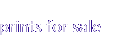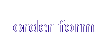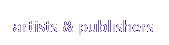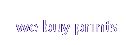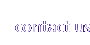# 123 = 4 = 567891011

######### 121. From Another World A(1957, 85 x 55 cm)### 122. From Another World B(1957, 85 x 55 cm)### 123. North Star(1957, 85 x 55 cm)### 124. South Star(1957, 78 x 55 cm)### 125. Black and Yellow(1957, 58 x 88 cm)### 126. Orion(1957, 72 x 52 cm)### 127. Andromeda(1957, 85 x 55 cm)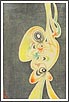### 128. Milky Way(1958, oban)### 129. Impression(1958, oban)### 130. Impression B(1958, oban)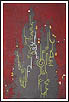### 131. Abstract Landscape C(1958, double oban)### 132. Image A(1958, double oban)### 133. Image B(1958, double oban)### 134. Image C(1958, double oban)### 135. Image D(1958, double oban)### 136. Image E(1958, double oban)### 137. Record of that Day(1958, 85 x 55 cm)### 138. Record of a Day(1958, 85 x 55 cm)### 139. Some Part in the Sky(1958, 85 x 55 cm)### 140. Both Side(1958, 85 x 55 cm)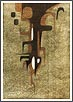### 141. Drop(1959, double oban)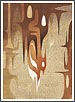### 142. Down(1959, double oban)### 143. Sound of the Moon(1959, double oban)### 144. Records of Every Days(1959, 82 x 53 cm)### 145. A Story A(1959, 85 x 54 cm)### 146. A Story B(1959, 54 x 85 cm)### 147. Image Mist(1960, oban)### 148. Image of a Day(1960, oban)### 149. Image in a Forest(1960, oban)### 150. Dreaming(1960, oban)### 151. Nebulae (lithograph)(1960, double oban)### 152. Color Proof(1960, double oban)### 153. Nebulae in Color (lithograph)(1960, double oban)### 154. Mystical (lithograph)(1960, double oban)### 155. Autumn(1960, 80 x 57 cm)### 156. Late Autumn(1960, 80 x 57 cm)### 157. History(1960, 54 x 85 cm)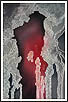### 158. Beginning(1960, 85 x 55 cm)### 159. Existence(1960, 85 x 55 cm)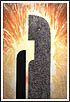# 123 = 4 = 567891011

######Malatya Web Tasarim Eskisehir Web Tasarim Youtube photos izmir haber